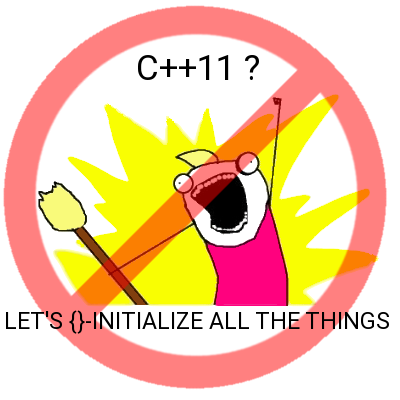# Let's not brace-initialize all the things

I was developing a small C++ library and I didn't have to consider supporting ancient compilers so I chose to embrace C++11. "Turn it up to 11".

It was a joy. I was finally able to use the auto keyword to make the code clearer. noexcept to help the compiler optimize. Even more compile-time optimization with constexpr. Lambdas instead for function pointers. Brace-initialization. move-constructors for efficient containers and values, and for solving the problem with non-copyable objects. =delete instead of the usual private-but-unimplemented constructors and assignment operators. using instead of typedef. Template specialization to deal with endianess.

All the good things.

## And then …

And then I hit a pitfall, which you can see in this much reduced example:

#include <vector>

class Value {
public:
using array_type = std::vector;

array_type array_elements;

Value()
{ }

Value(const Value &v)
{
*this = v;
}

Value(const array_type &a)
: array_elements{a}
{
}

Value& operator=(const Value &v) {
if(this!=&v) {
array_elements = v.array_elements;
}
return *this;
}

};

int main(void) {
Value v{Value::array_type{}};
}

Spot the bug

Hint: stack overflowThe problem is the brace-initialization:

: array_elements{a}

{…} is an initializer-list. Not a set of arguments for a constructor. An initializer-list can turn into arguments to a constructor. For simple things such as int, std::string and bool there are no surprises

int x{17};
std::string{"Hello world"};
bool b{false};

But consider this:

std::vector<int> v{17, 42, 117};

The compiler knows that {17, 42, 117} is an initializer-list. The compiler then decides what to do about that. In the line above it will decide to call std::vector<int>::vector(initializer_list...), which is what you'd expect and you end up with a nice vector with three elements in it. But with

array_elements{a}

the compiler will consider what to do with the initializer-list and go "hum... let's convert the single element in the initializer-list to a Value and then call std::vector<Value>::vector(initializer-list) with that. How do I convert the single element to a Value? Use the conversion constructor of course." And that is where the unexpected recursive call comes from, leading to a crash due to stack overflow

The GCC guys think that their interpretation of the standard and the above behaviour is correct. I tend to agree. Clang, Intel compiler, and MSVC don't. I suspect that it will get clarified at some point. Until then brace-initialization where user-defined conversion constructors are present is risky and the compiler could interpret the code in an unexpected way.

If it turns out that GCC is wrong then you still have to deal with until it gets fixed. If it turns out GCC is right then the other compilers will get fixed eventually, and your code will suddenly crash.

The solution is simple: Use normal parentheses

array_elements(a)

So let's not brace-initialize all the things?